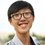# Chemistry - Mass Percentage

Problem Set

How do we express the concentration of a solute in a solution? We use Mass Percentage.

Mass Percentage is one of the way of representing the concentration of a solute in a solution. Mass percentage is calculated as the mass of a solvent divided by the total mass of the solution, multiplied by $100\%$.

So it is easy to find out the formula:

$\text{Mass Percentage }=\frac{m_{solute}}{m_{solution}}\times100\%$

A $200g$ beer contains $20g$ alcohol. So, the mass percentage of alcohol in the beer is:

$\frac{20g}{200g}\times100\%=10\%$.

Some other notes:

1. The mass percentage does not change with the temperature and air pressure.

2. There are other ways to express the concentration of a solute in a solution such as volume percentage, molality and amount-of substance concentration.

3. Some reference books express mass percentage as $g/g$. However, try not to use that to prevent a confusion with Solubility.

Now, there is an example:

I want to dilute a $50g$, $98\%$ $H_2SO_4$ to a $20\%$ solution. How much water should I add in grams to do this?

Solution:

$50g$ is the mass of solution.

$98\%$ is the mass percentage before adding water.

$20\%$ is the mass percentage after adding water.

Note that the mass of $H_2SO_4$ does not change before and after the water added.

So, let the fixed mass of $H_2SO_4$ be $c$ and the mass of water should add be $x$

$\frac{c g}{50g}\times100\%=98\%$

$c=\frac{98}{100}\times50$

$\frac{c g}{(50+x)g}\times100\%=20\%$

$c=\frac{20}{100}\times(50+x)$

Compare the two equations we have,

$\frac{98}{100}\times50=\frac{20}{100}\times(50+x)$

$x=195g$

So, the amount of water we should add is $195g$.Note by Christopher Boo
6 years, 6 months ago

This discussion board is a place to discuss our Daily Challenges and the math and science related to those challenges. Explanations are more than just a solution — they should explain the steps and thinking strategies that you used to obtain the solution. Comments should further the discussion of math and science.

When posting on Brilliant:

• Use the emojis to react to an explanation, whether you're congratulating a job well done , or just really confused .
• Ask specific questions about the challenge or the steps in somebody's explanation. Well-posed questions can add a lot to the discussion, but posting "I don't understand!" doesn't help anyone.
• Try to contribute something new to the discussion, whether it is an extension, generalization or other idea related to the challenge.

MarkdownAppears as
*italics* or _italics_ italics
**bold** or __bold__ bold
- bulleted- list
• bulleted
• list
1. numbered2. list
1. numbered
2. list
Note: you must add a full line of space before and after lists for them to show up correctly
paragraph 1paragraph 2

paragraph 1

paragraph 2

[example link](https://brilliant.org)example link
> This is a quote
This is a quote
    # I indented these lines
# 4 spaces, and now they show
# up as a code block.

print "hello world"
# I indented these lines
# 4 spaces, and now they show
# up as a code block.

print "hello world"
MathAppears as
Remember to wrap math in $$ ... $$ or $ ... $ to ensure proper formatting.
2 \times 3 $2 \times 3$
2^{34} $2^{34}$
a_{i-1} $a_{i-1}$
\frac{2}{3} $\frac{2}{3}$
\sqrt{2} $\sqrt{2}$
\sum_{i=1}^3 $\sum_{i=1}^3$
\sin \theta $\sin \theta$
\boxed{123} $\boxed{123}$

Sort by:

What does $20\%$ solution mean?

- 6 years, 6 months ago

Sorry, it is 20%

- 6 years, 6 months ago

In this particular example, it means that the mass of $H_2SO_4$ is $20$% of the mass of the solution.

- 6 years, 6 months ago

NICE POST!CAN YOU GIVE SOME NOTES ON EQUILIBRIUM.

- 6 years, 6 months ago

Ok but that may take some time, hope you can wait because now I am focusing on gases. :)

- 6 years, 6 months ago

I can't find any good image for this topic. If you found one, feel free to give me the link! For your information, I will not continue any notes involve solution such as molality, ideal solution, Raoult's Law etc. because this topics need the concept of mol. So, I will continue to those section after the idea of mol is presented. However, I will continue my chemistry notes in gas.

- 6 years, 6 months ago

Thanks for this note...Could you also start a series on Organic Chemistry, if possible. Looking forward to your note on mole concept.

- 6 years, 6 months ago

Oops, sorry I can't start Organic Chemistry because I am weak in that part.

- 6 years, 6 months ago